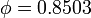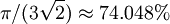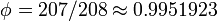# Difference between revisions of "Hard tetrahedron model"

The hard tetrahedron model is a subset of hard polyhedra model that has been put forward as a potential model for water.

## Maximum packing fraction

It has recently been shown that regular tetrahedra are able to achieve packing fractions as high as$\phi=0.8503$ (the hard sphere packing fraction is$\pi/(3 \sqrt{2}) \approx 74.048%$ ). This is in stark contrast to work as recent as in 2006, where it was suggested that the "...regular tetrahedron might even be the convex body having the smallest possible packing density".

## Truncated tetrahedra

Dimers composed of Archimedean truncated tetrahedra are able to achieve packing fractions as high as$\phi= 207/208 \approx 0.9951923$  while a non-regular truncated tetrahedra can completely tile space .